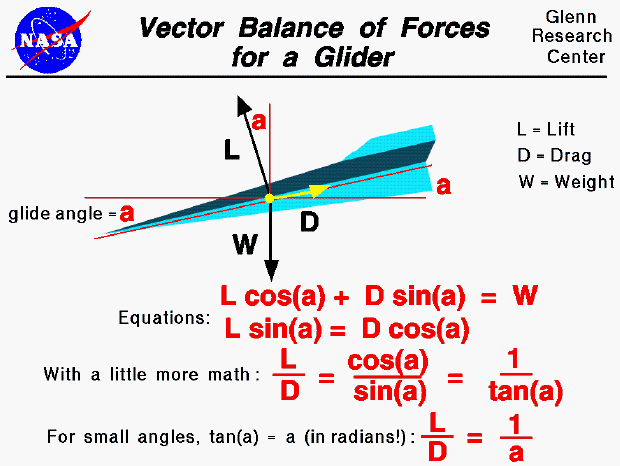This slide shows the balance of forces on a descending glider. A horizontal, thin red line has been drawn parallel to the ground and through the center of gravity. The flight path of the glider is along the other thin red line, which falls to the left. These two lines form an angle "a" called the glide angle.

The weight of the glider is given by the symbol "W" and is directed vertical, toward the center of the earth. The weight is then perpendicular to the horizontal red line drawn parallel to the ground. The drag of the glider is designated by "D" and acts along the flight path opposing the motion. Lift, designated "L," acts perpendicular to the flight path. Using some geometry theorems on angles, perpendicular lines, and parallel lines, we see the glide angle "a" also defines the angle between the lift and the vertical, and between the drag and the horizontal.

Assuming that the forces are balanced (no acceleration of the glider), we can write the two vector component equations for the forces. In the vertical direction, the weight (W) is equal to the lift (L) times the cosine (cos) of the glide angle (a) plus the drag (D) times the sine (sin) of the glide angle. In the horizontal direction, the lift (L) times the sine (sin) of the glide angle (a) equals the drag (D) times the cosine (cos) of the glide angle. Rearranging the horizontal component equation gives the relation that the lift divided by the drag is equal to the cosine of the glide angle divided by the sine of the glide angle. This ratio of trigonometric functions is equal to the cotangent of the angle, or the inverse of the tangent of the glide angle. For small angles, the tangent of the angle is almost the value of the angle in radians. This gives us the following relationship: the lift divided by the drag is equal to the inverse of the glide angle for small angles.

What good is all this for aircraft design? The lift divided by drag is called the L/D ratio and is an efficiency factor for aircraft. From the last equation we see that the higher the L/D, the lower the glide angle. The glide angle determines how far a glider will fly horizontally for each vertical foot (or meter) that it falls. The lower the glide angle, the farther the glider can fly. Therefore, designers of long distance gliders want a high L/D. We can also use measurements of the glide angle and weight of the glider to determine the L/D ratio as shown on another page.

Guided Tours